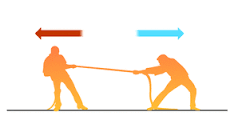## 4ESO Física i Química : ARTICLE 126 : FORCES AND DYNAMICS.

Publicado: 19/04/2017 10:30 por easyfisquim en 4ESO FiQ 2016-2017 ENGLISH (from article 47).- First of all we are gonna check a few important questions about forces in dynamics to understand what it means :

* ( Here Ill post several videos or more links about dynamics in the following days).

- Make sure that you study and look the following important ítems up :

1) Forces on an object.
2) Tension forcé.
3) Newton's First law.
4) Inertia and mass.
5) State of motion.
6) Balances and unbalanced forces.
7) Meaning of force.
8) Types of forces.
9) Drawing Free-body diagrams.
10) Determining the net force.
11) Newton's second law.
12) Finding acceleration.
13) Newton's third law.
14) Hooke's law.
15) Equilibrium.
16) Centre of gravity.
17) Weight.
18) Types of collision.

Comentarios  Ir a formulario